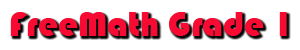Begin 1st Nine Weeks

1A

Identify Numbers 1 -5

1B

Identify Numbers 6 -10
Counting and Writing Numbers

1C

Numbers 0 - 5

1D

Numbers 6 - 10

1E

Numbers 11 - 20

1F

Numbers 11 - 20

Compare & Order Numbers

1G Write the Missing Numbers
1H

Which is More

1I

Which is Fewer

1J

Counting and Writing to 30

1K

Circle things that belong in the set

2A

Facts, Sums 0 to 2

2B

Facts, Sums 3 and 4

2C

Facts, Sums 5 and 6

2D

Picture Problems, In All

2E

2F

2G

2H

Order Numbers, Least to Greatest

Subtraction
3A

Subtraction, Cross Out to Subtract

3B

Subtraction From 1, 2, and 3

3C

Subtraction From 4 and 5

3D

Subtraction From 6

3E

Use Pictures to Write a Subtract. Sentence

3F

Subtraction, Picture Problems

3G

Subtraction Draw Pictures to Solve

4A

Subtraction From 7

4B

Subtraction From 8

4C

Subtraction From 9

4D

Draw Picture - Write a Subtraction Sentence

4E

Subtraction From 10

4F

Problem Solving, Subtraction, Draw Pictures

4G

Subtraction, Draw Pictures to Solve

5A

5B

5C

5D

Draw a Picture, Write Addition Sentence

6A

6B

6C

Subtraction, Draw Pictures to Solve

7A

7B

Fact Families to 10

7C

Draw a Picture, Write a Number Sentence

Graphs
8A

Picture Graphs, Read a Picture Graph

8B

Complete a Tally Table

8C

8D

Make a Bar Graph

9A

Place Value, Count by Tens

9B

Place Value - How Many Tens and Ones

9C

Place Value - Number that = Tens and Ones

9D

Place Value - Tens and Ones

9E

Counting & Writing to 100

Begin 2nd Nine Weeks

Inequality Symbols < > =

10A

Less Than <
10B

Greater Than >

10C

Inequality Symbols < > =

Number Order
10D Before, Between, After
10E

Count Forward & Backward

11A Number Patterns, Skip Count by 2s
11B Number Patterns, Skip Count by 5s
11C Number Patterns, Skip Count by 10s
11D Number Patterns, Even and Odd Numbers
11E Ordinal Numbers (1st - 2nd - 3rd etc)
12A Doubles and Doubles Plus One

12C

13A

13B

Fact Families, Write the #'s in Fact Family

13C

Sums and Differences to 12

13D

Find the Missing Addend, Related Facts

Geometry

14A

Three Dimensional Shapes, Cube

14B

3-D Shapes, Cylinder, Cone, Sphere

15A

Geometry, Symmetry

15B

Geometry, Slide and Turn

15C

Geometry, Congruent Shapes

16A

Geometry, Complete the Pattern

16B

Geometry, Make a Pattern

17A

17B

Add or Subt. Problem Solving Using Data

18A

Doubles Fact Families

18B

18C

18D

Subtraction Facts to18

Begin 3rd Nine Weeks

19A

19B

2 Digit subtract 1-digit

19C

2-digit subtract  2-digit

Fractions

20A

One Half

20B

One Fourth
20C One Third

20D

Color One Part, Circle the Fraction

Money
21A Pennies

21B

Pennies, Count Coins - Use Cent Symbol

21C

Nickels and Pennies, Write Amount

21D

Nickels and Pennies  use Cent Symbol

21E

Dimes and Pennies

21F

Price with Cent Symbol

21G

Dimes, Nickel and Pennies

21H

Quarters, Dimes, Nickels and Pennies

21I

Compare Coin Amounts

Time

22A

Time, Read a Clock, Time to the Hour

22B Time, Read a Clock, Time to the Half Hour
22C

Time, Draw Hands on a Clock

23A

Measurement

24A

Inches

24B

Print and Measure Length - Inches

24C

Centimeters

25A

Nonstandard Units

25B

Inches, Estimate and Measure

25C

Longest and Shortest

25D

Inches and Feet, Circle the Best Answer

25E

Centimeters, Estimate and Measure

25F

Circle the Object That is Heavier

25G

Circle the Object That is Lighter

26A

Pounds

26B

Kilograms

27A

Cup, Pint, Quart

27B

Temperature